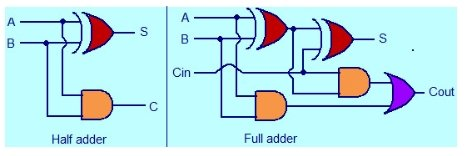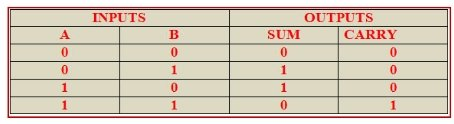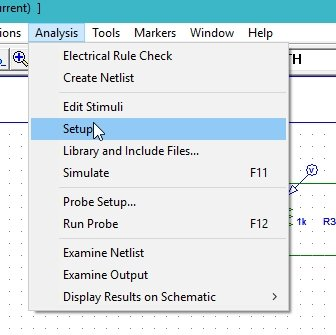# half adder and full adder simulation using PSpice : tutorial 13

In this tutorial I will explain you the working of a simple digital system known as an Adder. As the name implies an adder is used to add two or more digital numbers. At the start a brief and concise introduction to adder specifically a simplest half adder is provided with the explanation of the output they will show. After that the circuits is simulated using PSPICE and the result are compared with the theoretical discussion provided (which should be same). At the end of the tutorial you are provided with an exercise to do it by yourself, and in the next tutorials I will assume that you have done those exercises and I will not explain the concept regarding them.

### Introduction to Half and Full Adders

An adder is a digital circuit and as the name implies is used for addition of two or multiple numbers. A half adder is used to perform the addition between 2 numbers and if we are willing to add three numbers (digital) together than the adder used will be a full adder. A circuit diagram of half adder and full adder is shown in the figure below,Figure 1: Circuit diagrams

We should also take a glance on the truth tables of these adders. The truth table of half adder will have two inputs as shown in the truth table given below,Figure 2: Truth table of half adder

Below is given the truth table of a full adder which you can use to complete the exercise given at the end.Figure 3: Truth table of full adder

### Half adder simulation using PSpice

Figure 4: Opening the schematic

Figure 5: Saving schematic

Figure 6: Getting new part

Figure 7: Placing XOR gate

Figure 8: Placing AND gate

Figure 9: Placing digital supply

Figure 10: Placed components

Figure 11: Draw wire

Figure 12: Complete circuit diagram

Figure 13: Voltage marker

Figure 14: Placed voltage marker

Figure 15: Labeling

Figure 16: Labeled diagram

Figure 17: Input attributes

• The commands in the attributes window represents the value of the input at the corresponding time in the command before the space. The COMMAND1 = 0s 0 shows that at time 0s the value of the input dstm is 0 and the COMMAND2 = 1s 1 shows that the value will update to 1 at time 1 second. Similar is the case with COMMAND3 = 2s 0 that at 2 seconds the value of the input will be 0.
• Next step is to set the attributes of the input digital supply. Double click on the dstm2 which is used as the supply source B you connected in the circuit previously and set the input commands of the digital input system as shown in the figure below,Figure 18: Input attributes of B

Figure 19: Simulation setup

Figure 20: Transient properties

• The final time of the response is 2s because we have set the commands for only up to 2s. Now comes the simulation part, click on the analysis at the top bar of the schematic window and then click on simulate as shown in the figure below,

Figure 21: Simulation

Figure 22: Output of the adder

The output of the half adder is in accordance with the theoretical analysis as you can see from the figure above.

Exercise:

• Design and Simulate the full adder using the information provided in the introduction part and the procedure in the explanation portion